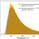FX:USOIL   CFDs on Crude Oil (WTI)
54 views
54
50 (fifty) is the natural number following 49 and preceding 51.

Fifty is the smallest number that is the sum of two non-zero square numbers in two distinct ways: 50 = 12 + 72 = 52 + 52. It is also the sum of three squares, 50 = 32 + 42 + 52, and the sum of four squares, 50 = 62 + 32 + 22 + 12.

The fifth magic number in nuclear physics.

In Kabbalah, there are 50 Gates of Wisdom (or Understanding)

Comment: * 50=1^2 + 7^2= 5^2 + 5^2
** 50= 6^2 + 3^2 + 2^2 + 1^2Just because everybody buys price does not have to go up.